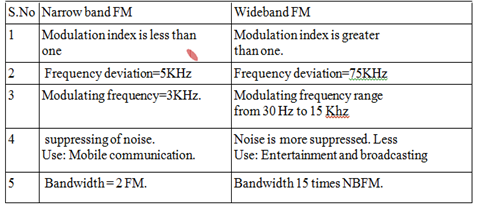### Communication Theory–Angle Modulation Systems (Unit 2)–Two Marks

Anna University

147402: Communication Theory

SEM / YEAR: IV/ II

QUESTION BANK

UNIT II

ANGLE MODULATION SYSTEMS

PART A

1. Compare narrow band FM and Wideband FM.

Ans:2. What is pre-emphasis? Why is it used?

Ans:

The premodulation filtering in the transistor, to raise the power spectral density of the base band signal in its upper-frequency range is called pre emphasis (or pre distortion) Pre emphasis is particularly effective in FM systems which are used for transmission of audio signals.

3. Define phase modulation.

Ans:

It is a type of modulation, used in communication systems, in which the phase of a carrier wave is varied by an amount proportional to the instantaneous amplitude of the modulating signal.

4. What is Narrowband FM?

Ans:

Narrowband FM has only a single pair of significant sidebands.

5. What are the applications of phase locked loop?

Ans:Phase-locked loops are widely used in , radio, telecommunications,computer and other electronic applications.

6. State the frequency in an FM system is 500 Hz and modulating voltage is 3 V,modulation index is 60. Calculate maximum deviation and bandwidth.

Ans:

Modulation index m = δ/fm

60 = δ/ 500

δ = 60 x 500 = 30 kHz

Bandwidth BW = 2 δ = 2 x 30 = 60 kHz.

7. Mention advantages of angle modulation over amplitude modulation.

Ans:

1. The amplitude of FM is constant. It is independent of depth of modulation.

Hence transmitter power remains constant in FM whereas it varies in AM.

2. Since amplitude of FM is constant, the noise interference is minimum in FM.

3. FM uses UHF and VHF ranges, the noise interference is minimum compared to AM which uses MF and HF ranges.

8. A 80MHz carrier is frequency modulated by sinusoidal signal of 1V amplitude and the frequency sensitivity is 100Hz/V. Find the approximate bandwidth of the FM waveform if the modulating signal has a frequency of 10KHz.

Ans:

2 ( δ + fm ) = 2 ( 100 + 10000 ) = 20.2 kHz.

9. What is frequency deviation in FM?

Ans:

Frequency deviation is the change in frequency that occurs in the carrier when it is acted on by a modulating signal frequency. The frequency deviation is typically given as the peak frequency shift in Hertz (∆f).

10. Differentiate FM and AM.

11. What is the bandwidth required for an FM wave in which the modulating frequency signal is 2 KHz and the maximum frequency deviation is 12 Khz?

Ans:

Bandwidth = 2 ( δ + fm ) = 2 ( 12 + 2 ) = 28 kHz.

12. A carrier wave of frequency 100 MHz is frequency modulated by a signal 20sin(200π x 103t) What is bandwidth of FM signal if the frequency sensitivity of the modulation is 25kHz/v.

Ans:

Bandwidth = 2 ( δ + fm ) = 2 ( 500 + 100 ) = 1.2 MHz.